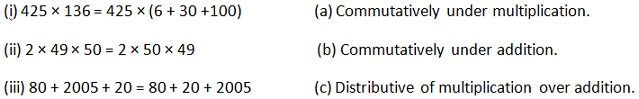Study Materials

# NCERT Solutions for Class 6th Mathematics

Page 2 of 3

## Chapter 2. Whole Numbers

### Exercise 2.2

#### Exercise-2.2

Q1. Find the sum by suitable rearrangement:

(a) 837 + 208 + 363

Solution: 837 + 208 + 363

= (837 + 208) + 363

= 1045 + 363

= 1408

Or Second Method

= 837 + 363 + 208

= (837 + 363) + 208

= 1200 + 208

= 1408

(b) 1962 + 453 + 1538 + 647

Solution:  1962 + 453 + 1538 + 6477

= (1962 + 453) + (1538 + 647)

= 2415 + 2185

= 4600

Or Second Method

= 1962 + 453 + 1538 + 6477

= (1538 + 1962) + (453 + 647)

= 3500 + 1100

= 4600

Q2. Find the product by suitable rearrangement:

(a) 2 × 1768 × 50

(b) 4 × 166 × 25

(c) 8 × 291 × 125

(d) 625 × 279 × 16

(e) 285 × 5 × 60

(f) 125 × 40 × 8 × 25

Solution:

(a) 2 x 1768 x 50

Solution:

2 x 50 x 1768

= (2 x 50) x 1768

= 100 x 1768

= 176800

(b) 4 x 166 x 25

Solution:

4 x 25 x 166

= (4 x 25) x 166

= 100 x 166

= 16600

(c) 8 x 291 x 125

Solution:

8 x 125 x 291

= 1000 x 291`

= 291000

(d) 125 x 40 x 8 x 25

Solution:

125 x 8 x 40 x 25

= (125 x 8) x (40 x 25)

= 1000 x 1000

= 1000000

(e) 285 × 5 × 60

Solution:

285 × 5 × 60

= 285 × (5 × 60)

= 285 × 300

= 85500

(f) 125 × 40 × 8 × 25

Solution:

125 × 40 × 8 × 25

= (125 × 8) × (40 × 25)

= 1000 × 1000

= 1000000

Q3. Find the value of the following:

(a) 297 × 17 + 297 × 3

Solution:

297 x (17 + 3)

= 297 x 20

= 5940

(b) 54279 × 92 + 8 × 54279

Solution:

54279 x (92 + 8)
= 54279 x 100

= 5427900

(c) 81265 × 169 – 81265 × 69

Solution:

81265 × 169 – 81265 × 69

= 81265 (169 – 69)

= 81265 (100)

= 8126500

(d) 3845 × 5 × 782 + 769 × 25 × 218

Solution:

3845 × 5 × 782 + 769 × 25 × 218

= 3845 × 5 × 782 + 769 × 5 × 5 × 218

3845 × 5 × 782 + 3845 × 5 × 218

= 3845 × 5 (782 + 218)

= 19225 × 1000

= 19225000

Q4. Find the product using suitable properties.

(a) 738 × 103

(b) 854 × 102

(c) 258 × 1008

(d) 1005 × 168

Solution:

(a) 738 × 103

= 738 (100 + 3)

= 738 × 100 + 738 × 3

= 73800 + 2214

76014

Solution:

(b) 854 × 102

= 854(100 + 2)

= 854 × 100 + 854 × 2

= 85400 + 1708

87108

Solution:

(c) 258 × 1008

= 258(1000 + 8)

= 258 × 1000 + 258 × 8

= 258000 + 2064

260064

Solution:

(d) 1005 × 168

= (1000 + 5) x 168

= 1000 x 168 + 5 x 168

= 168000 + 840

= 168840

5. A taxidriver filled his car petrol tank with 40 litres of petrol on Monday. The next day, he filled the tank with 50 litres of petrol. If the petrol costs Rs 44 per litre, how much did he spend in all on petrol?

Solution:  Taxi driver filled his car petrol tank on Monday = 40 L

He filled the petrol tank on Tuesday = 50 L

The petrol costs per liter = 44 Rs

He spend in all on petrol = (40 + 50) x 44

= 90 x 44

= 3960 Rs

6. A vendor supplies 32 liters of milk to a hotel in the morning and 68 liters of milk in the evening. If the milk costs Rs 15 per liter, how much money is due to the vendor per day?

Solution:  A vendor supplies milk to a hotel in the morning = 32 liters

=> A vendor supplies milk to a hotel in the evening = 68 liters

=> The cost of milk per liter = Rs 15

7. Match the following:Solution:

(i) ----------- (c)

(ii) ---------- (a)

(iii) ---------- (b)

Page 2 of 3

Chapter Contents: